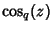## q-Factorial

The q-Analog of the Factorial (by analogy with the q-Gamma Function). Foran integer, the-factorial is defined byA reflection formula analogous to the Gamma Function reflection formula is given bywhereis the q-Cosine,is the q-Sine, andis q-Pi.

Gosper, R. W. Experiments and Discoveries in-Trigonometry.'' Unpublished manuscript.